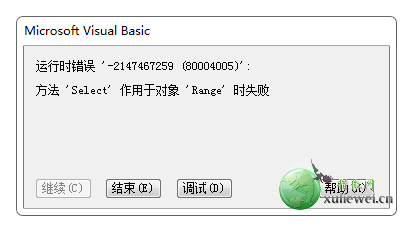# 方法’Select’作用于对象’Range’时失败的解决方法

2016-03-21 23:26 阅读 2,347 次 评论关闭

Excel VBA功能需求：

Excel VBA代码详情：

Private Sub CommandButton1_Click()
Dim r As Integer, x As String, xr As Integer, y As String

r = 2

Do While r < 84
Workbooks("工资管理系统.xls").Sheets("工人信息").Select

x = Workbooks("工资管理系统.xls").Sheets("工人信息").Cells(r, 5).Value
Workbooks("人事动态登记表.xlsx").Sheets("12月").Select
Sheets("12月").Cells(2, 4).Select
Set C = Range("A:F").Find(x, After:=Selection, LookIn:=-4176, LookAt:=xlWhole, SearchOrder:=xlByRows, SearchDirection:=xlNext, MatchCase:=True, MatchByte:=True, SearchFormat:=True)
If Not C Is Nothing Then
C.Select
xr = Selection.Row
End If
y = Workbooks("人事动态登记表.xlsx").Sheets("12月").Cells(xr, 3).Value

Workbooks("工资管理系统.xls").Sheets("工人信息").Select

Workbooks("工资管理系统.xls").Sheets("工人信息").Cells(r, 2).Value = y
r = r + 1
Loop

End SubPrivate Sub CommandButton1_Click()
Dim r As Integer, x As String, xr As Integer, y As String

r = 2

Do While r < 84
Workbooks("工资管理系统.xls").Sheets("工人信息").Select

x = Workbooks("工资管理系统.xls").Sheets("工人信息").Cells(r, 5).Value
Workbooks("人事动态登记表.xlsx").Sheets("12月").Select
Sheets("12月").Cells(2, 4).Select
Set C =Sheets("12月").Range("A:F").Find(x, After:=Selection, LookIn:=-4176, LookAt:=xlWhole, SearchOrder:=xlByRows, SearchDirection:=xlNext, MatchCase:=True, MatchByte:=True, SearchFormat:=True)
If Not C Is Nothing Then
C.Select
xr = Selection.Row
End If
y = Workbooks("人事动态登记表.xlsx").Sheets("12月").Cells(xr, 3).Value

Workbooks("工资管理系统.xls").Sheets("工人信息").Select

Workbooks("工资管理系统.xls").Sheets("工人信息").Cells(r, 2).Value = y
r = r + 1
Loop

End Sub